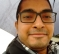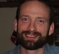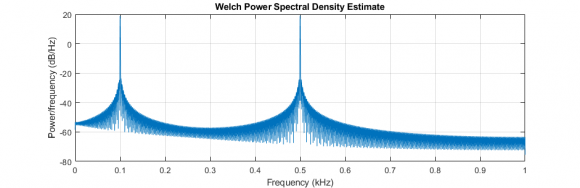## Generating Sine wave in C and Pwelch PlotStarted by 3 years ago2 replieslatest reply 3 years ago1776 views

I have made a C code to generate sine wave. I generate two sine waves of frequency 100 and 500 Hz respectively. The sampling rate is 2000 Hz.

But when I see the dumped data in frequency domain in MATLAB, the peaks are at wrong positions. Where I am doing wrong.

I use the following command in MATLAB

pwelch(sinewave.VarName1,[],[],[],Fs);

Here is the code. It can be compiled in any C compiler. I used codeblocks. Upon running it will dump three .dat files named sine_wave.dat, sine_wave_1.dat and sine_wave_2.dat which have sine waves of frequency 100, 500 and when both sinusoids are simply added.

#include<stdio.h>

#include<math.h>

#define sine_length 20000

#define sine_freq   100

#define sine_freq_1 500

int main()

{

double samp_rate = 2000; // sampling rate

double samp_time = (double)1/samp_rate; // sampling time

double pi = 3.14159;

double sineWV[sine_length]; // array for first sine wave

double sineWV_1[sine_length]; // array for second size wave

double sineWV_2[sine_length]; // array for combined sine wave

double theta = 0; // theta for first sine wave

double theta_1 = 0; // theta for second sine wave

FILE  *sine_wv_dump, *sine_wv_dump_1, *sine_wv_dump_2; // file pointer to store combined sine wave

sine_wv_dump = fopen("sine_wave.dat","w");

sine_wv_dump_1 = fopen("sine_wave_1.dat","w");

sine_wv_dump_2 = fopen("sine_wave_2.dat","w");

for(int i = 0; i < sine_length; i++)

{

theta       = 2*pi*sine_freq*i*samp_time*(pi/180);

theta_1     = 2*pi*sine_freq_1*i*samp_time*(pi/180);

sineWV[i]   = 10*(double)sin((double)theta);

sineWV_1[i] = 10*(double)sin((double)theta_1);

sineWV_2[i] = (double)sineWV[i] + (double)sineWV_1[i];

fprintf(sine_wv_dump, "\n%lf", sineWV[i]);

fprintf(sine_wv_dump_1, "\n%lf", sineWV_1[i]);

fprintf(sine_wv_dump_2, "\n%lf", sineWV_2[i]);

}

fclose(sine_wv_dump);

fclose(sine_wv_dump_1);

fclose(sine_wv_dump_2);

return 0;

}

[ - ]Try these changes:

1. theta       = 2.0*pi*sine_freq*(double)i*samp_time;

Note that nothing was in degrees to begin with, so the multiplication by pi/180 was incorrect. Also, it is cleaner in my opinion to explicitly cast all integers to double, so you're not relying on the math library to pick the right function/cast order. I also changed 2 to 2.0 for this reason.

2. sineWV[i] = 10.0*sin(theta);

Theta is already double, it doesn't need to be cast. 10 should be 10.0.

In math.h 10.0 is double type and 10.0f is float type (32 bit by default). Similarly sin() returns a double and sinf() returns a float().

There are a lot of places you are casting something that is already a double, and not casting things that should be double, or using 10 where you should use 10.0.

Hope this helps!

[ - ]